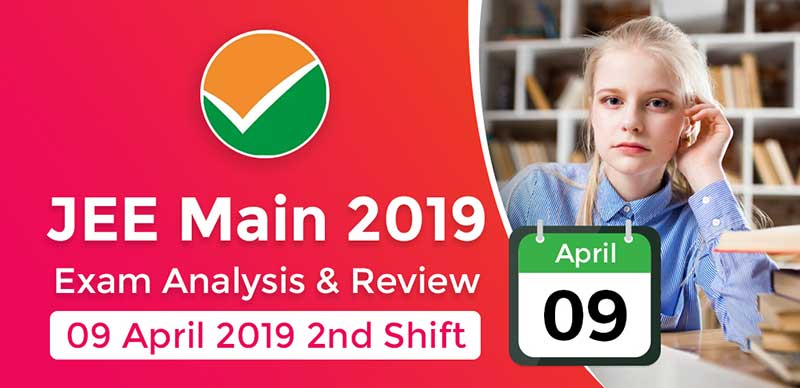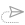### JEE Main 2019: Exam Analysis For Paper 1 (9 April - 2nd Shift)

The second shift of today’s examination was of an overall moderate in difficulty level. However, in this shift, the mathematics section was said to be the toughest by students. Physics was from easy to moderate as per most of the students. Chemistry was still the easiest of all.

JEE Main 2019 Paper 1: Exam Format

 Subject No of Questions Marks Mathematics 30 120 Chemistry 30 120 Physics 30 120 Total 90 360

Analysis for Physics:

There was more numerical based question in the physics section. More questions were there from 11th CBSE and were found to be tough.

Physics had 6 questions from mechanics and 3 from semiconductors. Also, questions from Relative motion, Convex mirrors were there in physics.

Analysis for Chemistry:

Chemistry section was easiest of all the three. Equal weightage was given to all organic, inorganic and physical chemistry.

There were more questions from organic chemistry Sn1 and Sn2 reactions. Also, the Stratosphere question was reported as the easiest question.

Analysis for Mathematics:

The mathematics section in this shift was the toughest of all. Although, Pattern and difficulty level is the same as the January session.

There were questions from coordinate geometry, 2D And 3D and calculus in maths. Also, the mathematics section had questions from Eclipse, parabola and trigonometry.

JEE Main 2019 Paper 1: Question & Answers

Maths

Qs.1: The length of the focal chord of parabola y square=16x through point P(1.4) on it is

Ans : 25

Qs.2: y=mx+7(3)^1/2 is a normal to the hyperbola (x^2)/24-(y^2)/18 . Then find the value of m?

Chemistry

Qs.1: Arrange in increasing order of oxidation state of the following: N2O, NO, NO2, N2O3, N2O5

Qs.2: Which is a path function?

Ans : heat and work

Qs.3: Floride present in which ore?

Qs.4: RMS speed of a gas at 1atm and 400k is 100m/s then what is the speed of gas at 2atm and 500K?

Qs.5: Aerosol is a colloidal solution of

Ans : solid in gas

Qs.6: In fullerene the structure consist?

Ans : 20-hexagonal and 12-pentagonal rings

Qs.7: Which of the following metal has large difference in its first and second ionization enthalpy a)Ba b) K c) Sr d) Ca

Ans : K

Qs.8: The linkage present in the units of a sucrose molecule is a) (1,2) b) (1.3) c) (1,4) d) (1,6)

Ans : -

Physics

Qs.1: A ring of radius R, disc of radius R/2 and a sphere of radius of R/4 are projected along the inclined plane upward, with the same velocity of center of mass. Assuming pur rolling throughout the motion, find the ratio of heights reached by them.

Ans : 20:15:14

Qs.2: A long current carrying wire having current I1 and a square loop of side carrying current I2 are lying in plane. Find the force on loop due to circle. -

Qs.3: A rope of length 'l', mass M is kept on smooth surface such that (1/n)th part of it is hanging from the surface. Find work done to lift rope on surface

Qs.4: A river is flowing with speed 2km/h. A swimmer who can swim with speed 4km/h in river want to cross the river. At what angle with river he should swim to reach the point directly opposite to the starting point. a)150 b) 90 c) 120 d) 60

Ans : 120

Qs.5: A simple pendulum of time period 'T' seconds, immerse in a liquid of density 1/16th of density of the bob of the pendulum. Find its new time period.

Ans : 4/√15T

Qs.6: The image of an object formed by a concave mirror of focal length 0.4m is errect and 5 times larger. Find the distance of object form in the mirror.

Ans : -0.32

Qs.7: I an YDSE, when we place a glass slob of refractive index μ in front of one of the slit. The Central maxima shifts by n-fringes. Find the thickness of glass slob (Wavelength of light used is λ)

Qs.8: A 5μF capacitor is given charge of 5μC. The its plates are moved slowly apart such that its new capacitance become 2μF. Find work done in the process

Student Reaction:

• There was a question in Chemistry in which students had to name the reaction for Phydroxyprophane + Bromide.
• There were no questions from semi-conductors.
• There was equal distribution of question in Chemistry from organic, and inorgani and physical
• Major topics in Physics was covered from Class 11th syllabi of NCERT.
• In chemistry, there was a question related to Carbonate ore.
• In Mathematics, there was one question in which the students had to find the value of sin 10, sin 30, sin 50.
• In Chemistry, there were questions related to the organic reaction, S1 and S2.
• There was a question in Maths in which the students were given values of CP and CV and they had to find the ratio.
• In physics, there were maximum numerical-type questions
• In Maths, there were questions from eclipse, parabola and trigonometry
• In Physics there were questions related to Convex mirror.
• There was one question each from Relative Motion and Sound & Frequency which was tricky.
• Students found Physics to be moderate and Chemistry difficult for Shift 2.
• In maths, there were maximum number of questions from 2-D, 3-D, Co-ordinate, Differential, Calculus.
• Maths was difficult for some students.
• Level of difficulty same as January attempt.

#### updates##### JEE Mains Pending Exam in August and NEET is Likely to Postponed, Click Here to Read More

23 June, 2021, 11:41 am##### JEE 2021 : Mathematics All-Time Most Difficult Previous Year Questions With Solutions

22 June, 2021, 11:06 am##### JEE 2021 : Chemistry All-Time Most Difficult Previous Year Questions With Solutions

21 June, 2021, 11:16 am##### JEE 2021 : List Of Students Most Desired Engineering Colleges In India Data According To NIRF Ranking

19 June, 2021, 10:53 am##### JEE 2021 : 10 JEE Main Practice Tests(60 MCQ+15 NAT) Based On 2021-New Pattern With Solution

17 June, 2021, 3:49 pm##### JEE 2021/2022 : COVID-19 Effect on JEE 2021 and 2022, Corona Impact on JEE Preparation, Important FAQs

16 June, 2021, 4:53 pmWe are providing something unique, useful and most importantly fun. By giving students a tool to find instant solutions to their doubts, we’re trying to make every student self-sufficient in practicing & completing their homework

### Follow Us

Copyright ©2021 All Rights Reserved, selfstudys.com×
Open Now